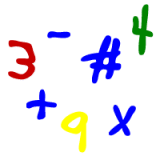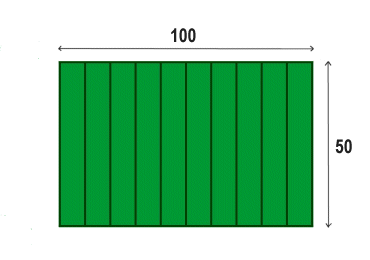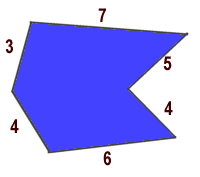Parents and Teachers: Support Ducksters by following us onor.

Kids Math

Finding the PerimeterSkills needed:
Multiplication
Subtraction
Polygons

The perimeter is the length around the outside of a polygon or the path that surrounds an area. This is different from the surface area. The surface area is how much surface is inside the polygon or space.

Let's show the difference between surface area and perimeter by looking at a football field. A football field is 100 yards long and around 50 yards wide. If you stayed right on the border and walked all the way around the football field you would walk 300 yards (see the picture). This is the perimeter.If you had to put down a tarp to cover the entire field so it wouldn't get wet, that would be the surface area. Go here to find out how to figure the surface area.

From the previous example we learned how to figure the perimeter of a rectangle. What we did was add each length twice and each width twice. If we say L = length, W= width, and P = perimeter, then we can have the following formula for the perimeter of a rectangle:

P = L + L + W + W or
P = 2xL + 2xW

A similar formula can be used for a square. Because all of the sides of a square are the same we can use L for all four sides. This means we figure the perimeter of a square as:

P = L + L + L + L or
P = 4xL

In general, to figure the perimeter of a polygon you just add up the length of the sides. The above two formulas are just short cuts where you can use multiplication because you know some of the sides are the same length.

Examples:

To figure the perimeter of the following triangle we use:P = a + b + c
P = 3 + 4 + 5
P= 12

Figure the perimeter for the following polygon:P = the sum of all the sides
P = 3 + 7 + 5 + 4 + 6 + 4
P = 29

Circles are a special case. We call the perimeter around a circle the circumference. This is a special formula:

Circumference = 2πr, where π = 3.14 and r = the radius of the circle

Go here for more on the geometry of the circle for kids.

Here are some perimeter formulas for different shapes for your reference:

 circle = 2πr where π = 3.14 and r = the radius triangle = a + b + c a, b, and c are the sides square = 4 x L L is the length of a side rectangle = 2 x L + 2 x W L = length and W = width general polygon = L1 + L2 + L3 + ….+ Ln L = length, n = number of sides

More Geometry Subjects

Circle
Polygons
Triangles
Pythagorean Theorem
Perimeter
Slope
Surface Area
Volume of a Box or Cube
Volume and Surface Area of a Sphere
Volume and Surface Area of a Cylinder
Volume and Surface Area of a Cone
Angles glossary
Figures and Shapes glossary

Back to Kids Math

Back to Kids StudyFollow us onor